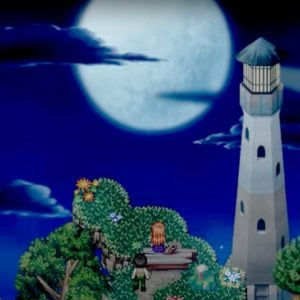# Kan R. Gao

1 Music Sheets

• ###### Genres:

Director & composer at Freebird Games (To the Moon, Finding Paradise, Impostor Factory).Credit: Wikipedia

## Artist's Music Sheets

•[d8] f [wd] f [ud] f [wd] f [d6] f [d0] f [td] f [d0] f [d4] f [d8] f [ed] f [d8] f [d5] f [d9] s [rd] f [g9] f [d8] f [wd] f [ud] f [wd] f [d6] f [d0] f [td] f [d0] f [d4] f [d8] f [ed] f [d8] f [d5] f [d9] s [rd] f [g9] f [d8] [wf] [tf] [uf] [of] u s h [s6]h 0 e [ts] u o t o [o4] p [s8] o [qp] s [d8] f [d5] f [d9] s [wd] f [rg] f [d8] [wf] [tf] [uf] [of] u s j [s6]j 0 [he] [ts] u s [td] s [s4] 8 q [oe] t o [ts] o [o5]s 9 w [ro]a y s a [s6] 0 e [ts]l|u d z [d3]z 7 [s0]l [wa]k r oh w o [o4]h 8| [pe]j|t| [p4]j 8 q [pe]j [tp]j|[a3]k| [s6]l 0 e [ts]l|u dz sl [a3]k 7 0 [wo]h|r jb| [j4]b 8 [qh]v e|t|gc|fx| [c8]wti[cO] c c [cH] x l h [wh]z r [ys] [od] [ywo]a y|o [o5]s d [f8] w t w u w [ts] [a9] [a6]f 0 e 0 t 0 [se] [a0] 5 h [h2] [j6] [h9] q [ge] y f d [a5] 7 [t9] y r o s d [f8] w t w u w [ts] [yd7] [f^] q [dE] q y q [ts] [d7] [d6]f 0 [se] r t u s d [a3] 7 0 w r u o a f|h a [p4] 8 [oe]p[o8]p[qo]p[o8]p[oe]p[o8]p [rqa95]|o o og f| s|d
Level: 6
Length: 01:36
Intermediate